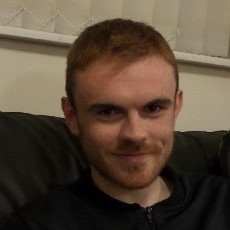# Dr Gareth Tracey MSci PhDSchool of Mathematics
Birmingham Fellow

## Contact details

School of Mathematics
Watson Building
University of Birmingham
Edgbaston
Birmingham
B15 2TT
UK

I am an EPSRC Postdoctoral Fellow and Birmingham Fellow in the School of Mathematics.

## Qualifications

• PhD in Mathematics, University of Warwick, 2017
• MSci in Mathematics, Maynooth University, 2013

## Biography

Before joining the School of Mathematics in 2021, Gareth held postdoctoral positions at the University of Oxford, the Alfréd Rényi Institute of Mathematics, and the University of Bath. His doctoral studies were at the University of Warwick under the supervision of Prof. Derek Holt, graduating in 2017.

I have a number of ideas for MSci and PhD projects in some of the areas in the research themes, so if you are interested, then please do get in touch!

## Research

### Research Themes

My primary area of research is Group Theory, and the following is a non-exhaustive list of the particular topics that I am interested in:

• Generation properties of finite groups.
• Representation theory, subgroup structure, and properties of automorphisms of finite almost simple groups.
• Permutation groups and associated combinatorics.
• Subgroup enumeration problems in finite and infinite groups.
• Probabilistic Group Theory.

### Research Activity

Gareth's current research seeks to build on a fascinating link which has emerged between a problem in Group Theory, and one of the most famous unsolved problems in mathematics: the Inverse Galois Problem.

Galois theory was discovered by the French mathematician Évariste Galois in the nineteenth century as a tool to study (integer) polynomial equations of degree greater than 4, and when they can be solved by radicals. To a set of roots of such a polynomial, Galois associated an algebraic structure which we now call a Galois group. This structure is a set together with a binary operation which preserves the symmetries in the roots of the polynomial, and studying this operation allows us to deduce properties of the roots. In this way, Galois developed a theory whereby one can translate questions about a very complicated polynomial equation to questions about its Galois group, which is often easier and more concise to study.

The Galois groups associated to integer polynomials are finite groups, but what about the other way around? Is every finite group the Galois group of some integer polynomial? This is called the "Inverse Galois Problem" (IGP), and a complete solution has evaded mathematicians for almost 200 years. A group which is the Galois group of an integer polynomial is said to "satisfy IGP". Some specific cases have been dealt with (the group of all symmetries of a finite set of size n - the symmetric group of degree n - is known to satisfy the IGP, for example), but even some relatively 'small' groups remain elusive.

In 2008, the number theorists Jouve, Kowlaski and Zywina announced a new technique to study the IGP in the Weyl groups of simple algebraic groups - an important class of groups in geometry. This spawned a renewed optimism for the IGP, and led to further developments of the technique by Andrea Lucchini and Gareth (using powerful group theoretic techniques) and the mathematicians Eberhard, Ford and Green (using powerful techniques from probability theory and combinatorics).

Gareth's current research programme seeks to build on these techniques by combining the group theoretic, probabilistic, and combinatorial approaches mentioned above. The specific problems he is working on range from answering important questions concerning these techniques in the finite simple groups, to answering some long-standing questions posed by B.L. van der Waerden concerning random invariable generation.

## Publications

### Recent publications

#### Article

Noce, M, Tracey, G & Traustason, G 2020, 'A left 3-Engel element whose normal closure is not nilpotent', Journal of Pure and Applied Algebra, vol. 224, no. 3, pp. 1092-1101.

Fernandez-Alcober, G, Noce, M & Tracey, G 2020, 'Engel elements in branch groups', Journal of Algebra, vol. 554, pp. 54-77.

Lucchini, A, Marion, C & Tracey, G 2020, 'Generating maximal subgroups of finite almost simple groups', Forum of Mathematics, Sigma, vol. 8, no. e32.

Guralnick, RM & Tracey, G 2020, 'On the generalized Fitting height and insoluble length of finite groups', Bulletin of the London Mathematical Society, vol. 52, no. 5, 8, pp. 924.

Lucchini, A & Tracey, G 2019, 'Finite groups with large Chebotarev invariant', Israel Journal of Mathematics, vol. 235, pp. 169-182.

Tracey, G 2019, 'Invariable generation of permutation and linear groups', Journal of Algebra, vol. 524, 40, pp. 250.

Tracey, G & Traustason, G 2018, 'Left 3-Engel elements in groups of exponent 60', International Journal of Algebra and Computation, pp. 673-695.

Tracey, G 2018, 'Minimal generation of transitive permutation groups', Journal of Algebra, vol. 509, pp. 40-100.

Lucchini, A & Tracey, G 2017, 'An upper bound on the Chebotarev invariant of a finite group', Israel Journal of Mathematics, vol. 219, no. 1, pp. 449-467.

Tracey, G 2016, 'Generating minimally transitive permutation groups', Journal of Algebra, vol. 460, pp. 380-386.